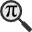## General

Display information for equation id:math.220515.27 on revision:220515

* Page found: Probability theory (eq math.220515.27)

(force rerendering)

Cannot find the equation data in the database. Fetching from revision text.

Occurrences on the following pages:

Hash: dc09544fdb33e535b6ba5350e797ac7e

TeX (original user input):

\Omega\,


TeX (checked):

\Omega \,


### LaTeXML (experimental; uses MathML) rendering

MathML (593 B / 287 B) :${\displaystyle\Omega\,}$
<math xmlns="http://www.w3.org/1998/Math/MathML" id="p1.1.m1.1" class="ltx_Math" alttext="{\displaystyle\Omega\,}" display="inline">
<semantics id="p1.1.m1.1a">
<mi mathvariant="normal" id="p1.1.m1.1.1a" xref="p1.1.m1.1.1.cmml">Ω</mi>
<annotation-xml encoding="MathML-Content" id="p1.1.m1.1b">
<ci id="p1.1.m1.1.1.cmml" xref="p1.1.m1.1.1">Ω</ci>
</annotation-xml>
<annotation encoding="application/x-tex" id="p1.1.m1.1c">{\displaystyle\Omega\,}</annotation>
</semantics>
[/itex]


SVG (1.186 KB / 701 B) :

### MathML with SVG or PNG fallback (recommended for modern browsers and accessibility tools) rendering

SVG image empty. Force Re-Rendering

SVG (0 B / 8 B) :

PNG (0 B / 8 B) :

$\Omega \,$## Translations to Computer Algebra Systems

### Translation to Maple

In Maple: Omega

\Omega: Could be The omega constant == the Adamchik constant.

But this system don't know how to translate it as a constant. It was translated as a general letter.

### Translation to Mathematica

In Mathematica: \[CapitalOmega]

\Omega: Could be The omega constant == the Adamchik constant.

But this system don't know how to translate it as a constant. It was translated as a general letter.

## Similar pages

Calculated based on the variables occurring on the entire Probability theory page

## Identifiers

• $\Omega$### MathML observations

0results

0results

no statistics present please run the maintenance script ExtractFeatures.php

0 results

0 results